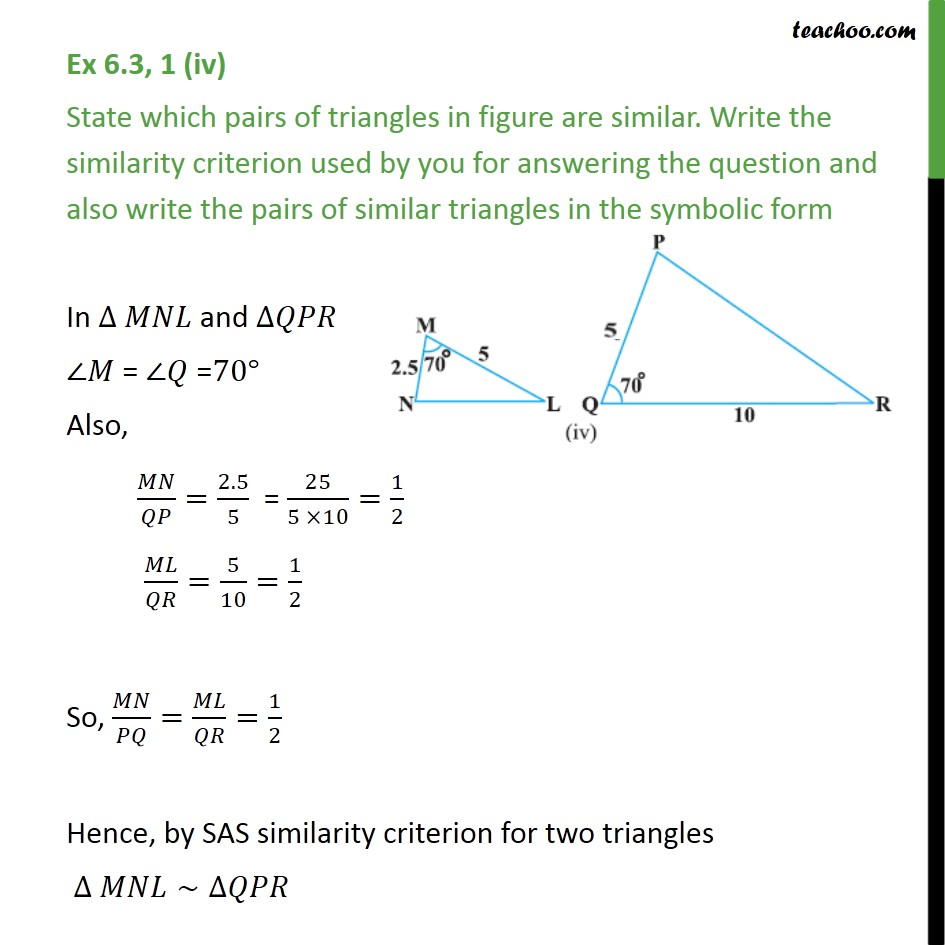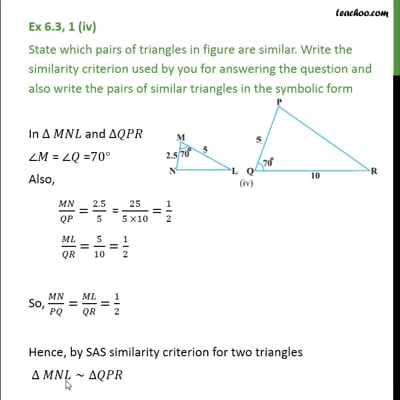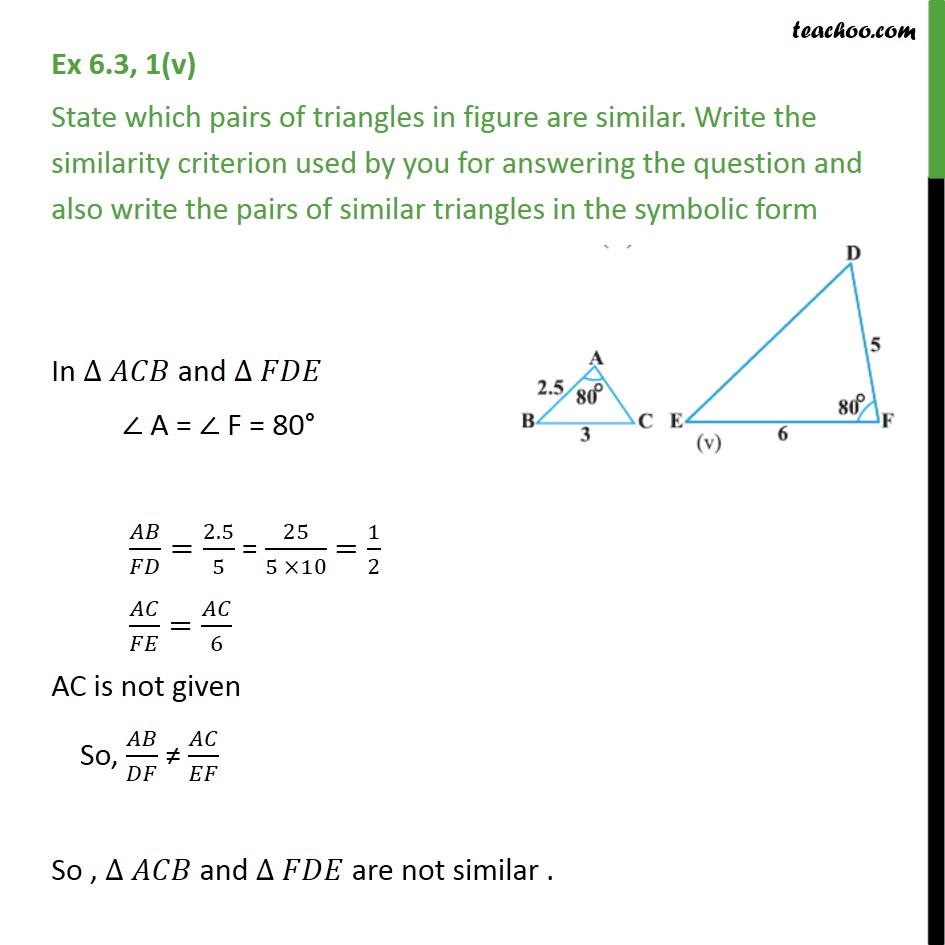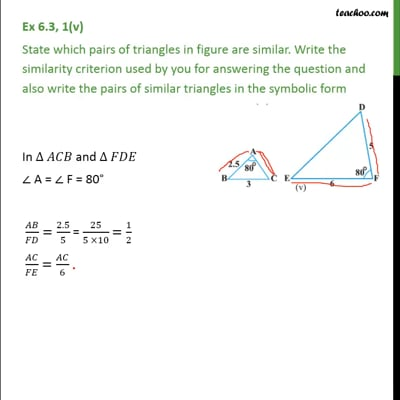SAS Similarity

Chapter 6 Class 10 Triangles
Concept wiseThis video is only available for Teachoo black usersThis video is only available for Teachoo black users

Introducing your new favourite teacher - Teachoo Black, at only ₹83 per month

### Transcript

Ex 6.3, 1 (iv) State which pairs of triangles in figure are similar. Write the similarity criterion used by you for answering the question and also write the pairs of similar triangles in the symbolic form In ∆ 𝑀𝑁𝐿 and ∆𝑄𝑃𝑅 ∠𝑀 = ∠𝑄 =70° Also, 𝑀𝑁/𝑄𝑃=2.5/5 = 25/(5 ×10)=1/2 𝑀𝐿/𝑄𝑅=5/10=1/2 So, 𝑀𝑁/𝑃𝑄=𝑀𝐿/𝑄𝑅=1/2 Hence, by SAS similarity criterion for two triangles ∆ 𝑀𝑁𝐿∼∆𝑄𝑃𝑅 Ex 6.3, 1(v) State which pairs of triangles in figure are similar. Write the similarity criterion used by you for answering the question and also write the pairs of similar triangles in the symbolic form In ∆ 𝐴𝐶𝐵 and ∆ 𝐹𝐷𝐸 ∠ A = ∠ F = 80° 𝐴𝐵/𝐹𝐷=2.5/5 = 25/(5 ×10) =1/2 𝐴𝐶/𝐹𝐸=𝐴𝐶/6 AC is not given So, 𝐴𝐵/𝐷𝐹 ≠ 𝐴𝐶/𝐸𝐹 So , ∆ 𝐴𝐶𝐵 and ∆ 𝐹𝐷𝐸 are not similar .